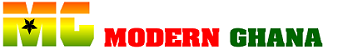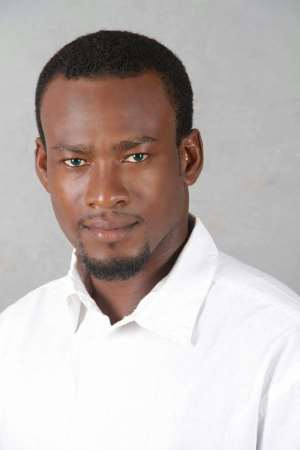20.01.2017 Opinion

# Ghana Education Is Sick ; Who Carries The Blame?Posterity will castigate us mercilessly especially the stakeholders in the education sector if we fail to address issues hampering teaching and learning in the country.

Let me state unequivocally that , Ghana Education is sick ; and who we should we blame for causing this dreadful sickness?

Well, i surmise it a high time we halt the blame game and rather provide concrete solution to this dreadful sick we are crying over.

To be candid, as a country in order to enhance the education sector, there is a need for the Ministry of Education, the Teachers Associations of Ghana and some Publishers to take a critical look at some of our textbooks.

This is because we realized that some of the books being use for teaching in our schools contain dreadful errors.

It is in this regard, a Non- Governmental Organization called ACHIEVERS EDUCATION TV, took a swift action to inform the general public,stakeholders as well as to all the authorities concern, to take a critical look at “three of the common mistakes “ in the Ghanaian classroom education.

In Ghanaian schools, it is taught that, in the order of operation of Arithmetics, the ‘O’ in ‘BODMAS’ stands for ‘OF’ which is to mean multiplication.

So we here students recite, “Bracket Of Division, Multiplication, Addition and Subtraction.” We are sorry to say that is no small lie.

‘BODMAS’ is an acronym which rightly stands for Brackets, Order, Division, Multiplication, Addition, and Subtraction. ‘O’ in ‘BODMAS’ stands for ‘ORDER’ but not ‘OF’. Order means exponent (index) but not multiplication as it is taught in our schools.

This error is one of the many reasons for the poor grades and the fear of Mathematics by many students. Not knowing that the ‘O’ stands for ‘ORDER’, which also means exponent or index, makes it difficult for students to do arithmetic operations with ease.

Secondly, in Ghana, the number 125,000 is commonly read as ‘one hundred and twenty five thousands.

This is wrong because, in reading numbers, the word ‘AND’ is use for reading decimal numbers to mean a decimal point. So the number 125,000 is rightly read as ‘one hundred twenty five thousand.’ The number 100.025 is rightly read as ‘hundred and twenty five thousandths. 202 is two hundred two, not two hundred and two. 200.2 is read rightly read as two hundred and two tenths. So the word ‘AND’ should only be used when reading decimal numbers to mean a decimal point.

Thirdly, when a Ghanaian student says ‘Almighty formula’ he/she is referring to [endif]--> It is rightly called the ‘Quadratic Formula’. Any mathematical expression which can be written as [endif]-->+[endif]--> (where [endif]--> is call a quadratic expression. When it is equated to zero, it becomes a quadratic equation ([endif]-->+[endif]-->. When [endif]--> is made the subject of the equation, we get [endif]--> [endif]--> , which is call the Quadratic Formula. When we asked fifty S.H.S students to write the quadratic formula, they said they had no idea. However, when we asked them to write the almighty formula, they wrote the quadratic formula.

References to the above assertions can be made from https://www.reference.com>maths>BODMAS